# ML Aggarwal Class 7 Solutions for ICSE Maths Chapter 6 Ratio and Proportion Objective Type Questions

## ML Aggarwal Class 7 Solutions for ICSE Maths Chapter 6 Ratio and Proportion Objective Type Questions

Mental Maths

Question 1.
Fill in the blanks:
(i) The simplest form of the ratio $$\frac { 1 }{ 6 }$$ : $$\frac { 1 }{ 4 }$$ is ………
(ii) 75 cm : 1.25 m = ……..
(iii) If two ratios are equivalent, then the four quantities are said to be in ………
(iv) If 8, x, 48 and 18 are in proportion then the value of x is ………
(v) If the cost of 10 pencils is ₹ 15, then the cost of 6 pencils is ……..
(vi) If a cyclist is travelling at a speed of 15 km/h, then the distance covered by him in 20 minutes is ……..
Solution:Question 2.
State whether the following statements are true (T) or false (F):
(i) A ratio is always greater than 1.
(ii) Ratio of half an hour to 20 seconds is 30 : 20.
(iii) The ratio 5 : 7 is greater than the ratio 5 : 6.
(iv) If the numbers 3, 5, 12 and x are in proportion then the value of x is 20.
(v) Ratio 3 : 2 and 4 : 5 are equivalent.
Solution: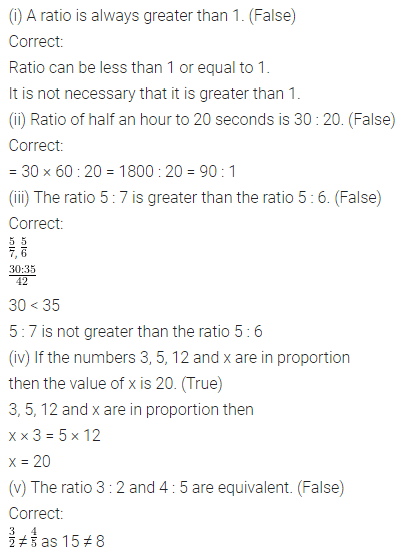Multiple Choice Questions

Choose the correct answer from the given four options (3 to 14):
Question 3.
A ratio equivalent to 6 : 10 is
(a) 3 : 4
(b) 18 : 30
(c) 12 : 40
(d) 5 : 3
Solution: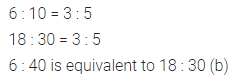Question 4.
A ratio equivalent to the ratio $$\frac { 2 }{ 3 }$$ : $$\frac { 3 }{ 4 }$$ is
(a) 4 : 6
(b) 8 : 9
(c) 6 : 8
(d) 9 : 8
Solution: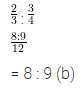Question 5.
The ratio of 75 mL to 3 litres is
(a) 25 : 1
(b) 40 : 1
(c) 1 : 40
(d) 3 : 200
Solution:Question 6.
The ratio of the number of sides of a rectangle to the number of edges of a cuboid is
(a) 1 : 2
(b) 1 : 3
(c) 2 : 3
(d) none of these
Solution: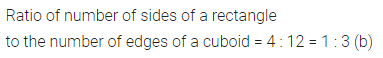Question 7.
In a class of 35 students, the number of girls is 20. The ratio of number of boys to the number of girls in the class is
(a) 3 : 4
(b) 4 : 3
(c) 7 : 4
(d) 7 : 3
Solution:Question 8.
The ratio of number of girls to the number of boys in a class is 6 : 7. If there are 21 boys in the class, then the number of girls in the class is
(a) 39
(b) 24
(c) 18
(d) 13
Solution: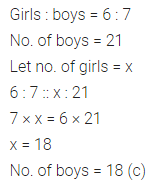Question 9.
Two numbers are in the ratio 3 : 5. If the sum of the numbers is 144, then the largest number is
(a) 48
(b) 54
(c) 72
(d) 90
Solution:Question 10.
If x, 12, 8 and 32 are in proportion, then x is
(a) 6
(b) 4
(c) 3
(d) 2
Solution: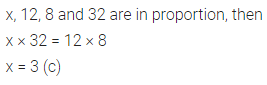Question 11.
If 3, 12 and x are in continued proportion, then x is
(a) 4
(b) 6
(c) 16
(d) 48
Solution:Question 12.
If the weight of 5 bags of sugar is 27 kg, then the weight of one bag of sugar is
(a) 5.4 kg
(b) 5.2 kg
(c) 5.4 kg
(d) 5.6 kg
Solution: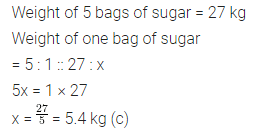Question 13.
Sonali bought one dozen notebooks for ₹ 66. What did she pay for one notebook?
(a) ₹ 6.50
(b) ₹ 6.60
(c) ₹ 5.60
(d) ₹ 5.50
Solution: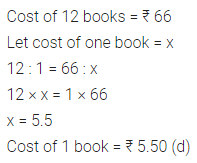Question 14.
The speed of 90 km/h is equal to
(a) 10 m/sec
(b) 18 m/sec
(c) 25 m/sec
(d) none of these
Solution:Value Based Questions

Question 1.
Sudhanshu divided his property into two parts in the ratio 8 : 5. If the first part is ₹ 1,60,000 and second part is donated to an orphanage, find the amount donated to the orphanage. What values are being promoted?
Solution:Higher Order Thinking Skills (HOTS)

Question 1.
Present ages of Rohit and Mayank are in the ratio 11 : 8. 8 years hence the ratio of their ages will be 5 : 4. Find their present ages.
Solution:Question 2.
Ratio of length and breadth of a rectangle is 3 : 2. If the length of the rectangle is 5 m more than the breadth, find the perimeter of the rectangle.
Solution: